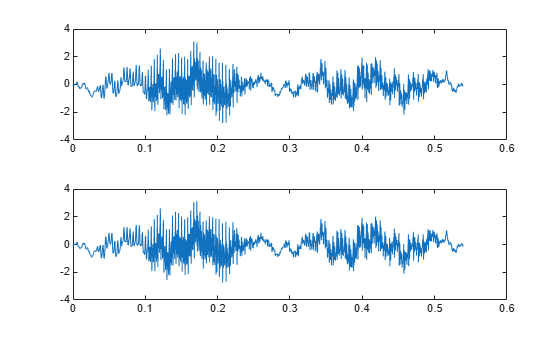# Change Signal Sample Rate

This example shows how to change the sample rate of a signal. The example has two parts. Part one changes the sample rate of a sinusoidal input from 44.1 kHz to 48 kHz. This workflow is common in audio processing. The sample rate used on compact discs is 44.1 kHz, while the sample rate used on digital audio tape is 48 kHz. Part two changes the sample rate of a recorded speech sample from 7418 Hz to 8192 Hz.

Create an input signal consisting of a sum of sine waves sampled at 44.1 kHz. The sine waves have frequencies of 2, 4, and 8 kHz.

```Fs = 44.1e3; t = 0:1/Fs:1-1/Fs; x = cos(2*pi*2000*t) + 1/2*sin(2*pi*4000*(t-pi/4)) + ... 1/4*cos(2*pi*8000*t);```

To change the sample rate from 44.1 to 48 kHz, you have to determine a rational number (ratio of integers), `P/Q`, such that `P/Q` times the original sample rate, 44100, is equal to 48000 within some specified tolerance.

To determine these factors, use `rat`. Input the ratio of the new sample rate, 48000, to the original sample rate, 44100.

```[P,Q] = rat(48e3/Fs); abs(P/Q*Fs-48000)```
```ans = 7.2760e-12 ```

You see that `P/Q*Fs` only differs from the desired sample rate, 48000, on the order of $1{0}^{-12}$.

Use the numerator and denominator factors obtained with `rat` as inputs to `resample` to output a waveform sampled at 48 kHz.

`xnew = resample(x,P,Q);`

If your computer can play audio, you can play the two waveforms. Set the volume to a comfortable level before you play the signals. Execute the `sound` commands separately so that you can hear the signal with the two different sample rates.

```% sound(x,44100) % sound(xnew,48000)```

Change the sample rate of a speech sample from 7418 Hz to 8192 Hz. The speech signal is a recording of a speaker saying "MATLAB®".

`load mtlb`

Loading the file `mtlb.mat` brings the speech signal, `mtlb`, and the sample rate, `Fs`, into the MATLAB workspace.

Determine a rational approximation to the ratio of the new sample rate, 8192, to the original sample rate. Use `rat` to determine the approximation.

`[P,Q] = rat(8192/Fs);`

Resample the speech sample at the new sample rate. Plot the two signals.

```mtlb_new = resample(mtlb,P,Q); subplot(2,1,1) plot((0:length(mtlb)-1)/Fs,mtlb) subplot(2,1,2) plot((0:length(mtlb_new)-1)/(P/Q*Fs),mtlb_new)```If your computer has audio output capability, you can play the two waveforms at their respective sample rates for comparison. Set the volume on your computer to a comfortable listening level before playing the sounds. Execute the `sound` commands separately to compare the speech samples at the different sample rates.

```% sound(mtlb,Fs) % sound(mtlb_new,8192)```